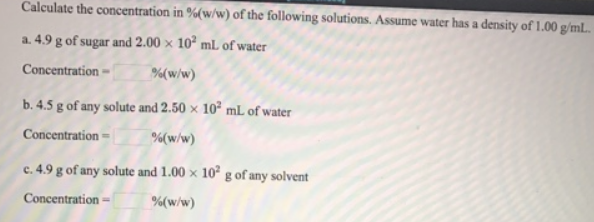# Problem: Calculate the concentration in %(w/w) of the following solutions. Assume water has a density of 1.00 g/mL. a. 4.9 g of sugar and 2.00 x 10 2 mL of water b. 4.5 g of any solute and 2.50 x 10 2 mL of water c. 4.9 g of any solute and 1.00 x 10 2 g of any solvent

###### FREE Expert Solution
99% (54 ratings)###### Problem Details

Calculate the concentration in %(w/w) of the following solutions. Assume water has a density of 1.00 g/mL.

a. 4.9 g of sugar and 2.00 x 10 2 mL of water

b. 4.5 g of any solute and 2.50 x 10 2 mL of water

c. 4.9 g of any solute and 1.00 x 10 2 g of any solventFrequently Asked Questions

What scientific concept do you need to know in order to solve this problem?

Our tutors have indicated that to solve this problem you will need to apply the Mass Percent concept. You can view video lessons to learn Mass Percent. Or if you need more Mass Percent practice, you can also practice Mass Percent practice problems.